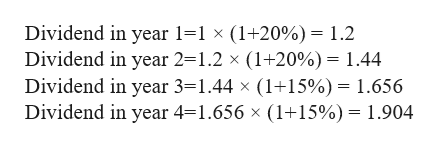# Babbalu Inc. just paid a dividend of \$1 per share. Babbalu expects to increase its annual dividend by 20% per year for the next two years and by 15% per year for the following two years. Starting in year 5, Babbalu is expects to pay a constant annual dividend of \$3 a share. What is the current value of this stock if the required rate of return is 12%?\$17.71\$18.97\$20.50\$21.08

Question
343 views

Babbalu Inc. just paid a dividend of \$1 per share. Babbalu expects to increase its annual dividend by 20% per year for the next two years and by 15% per year for the following two years. Starting in year 5, Babbalu is expects to pay a constant annual dividend of \$3 a share. What is the current value of this stock if the required rate of return is 12%?

\$17.71
\$18.97
\$20.50
\$21.08
check_circle

Step 1

Given that the dividend just paid is \$1.
The dividend growth is expected to be 20% per year for next 2 years and 15% per year for the following two years. Hence, the dividend payment is calculated as:

Step 2help_outlineImage TranscriptioncloseDividend in year 1=1 x (1+20%)= 1.2 Dividend in year 2=1.2 x (1+20%)= 1.44 Dividend in year 3-1.44 x (1+15%) = 1.656 Dividend in year 4=1.656 x (1+15%)= 1.904 fullscreen
Step 3

Now, the price beyond year 4 (starting from year 5) when the expected c...

### Want to see the full answer?

See Solution

#### Want to see this answer and more?

Solutions are written by subject experts who are available 24/7. Questions are typically answered within 1 hour.*

See Solution
*Response times may vary by subject and question.
Tagged in